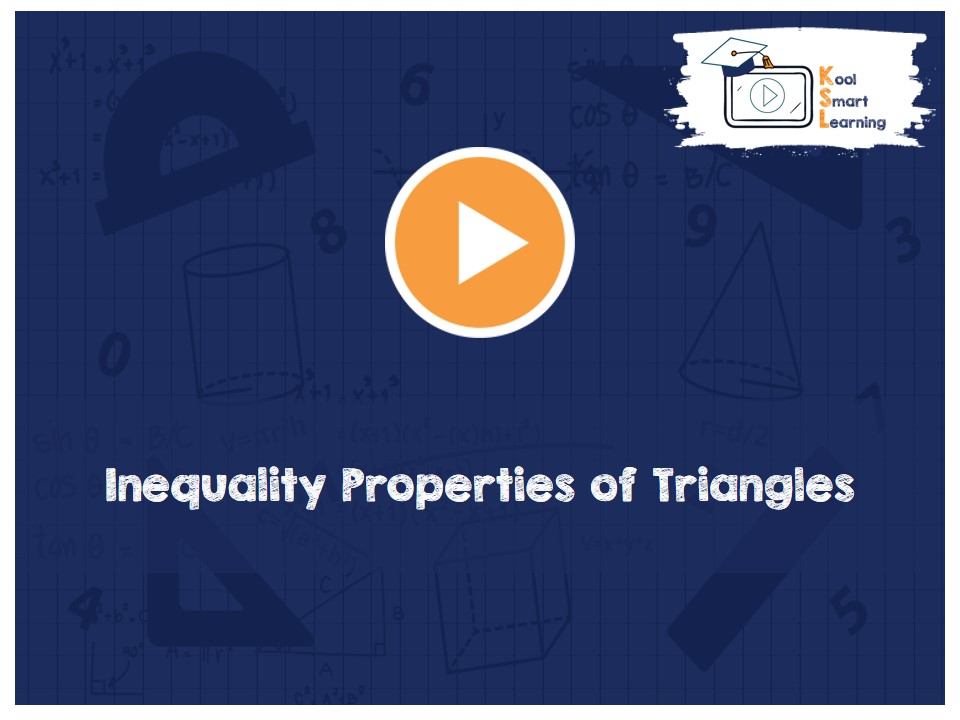At KoolSmartLearning, we intend to harness the power of online education to make learning easy.## Inequality Properties of Triangles

/  Inequality Properties of Triangles## Inequality Properties of Triangles

This video talks about the theorems related to the different properties of triangles. The proof of each theorem is also explained. The properties explained in this video are – If two sides of a triangle are unequal, the angle opposite larger side is greater, its converse theorem (In a triangle, the side opposite the greater angle is larger) and the sum of any two sides of a triangle is greater than the third side of the triangle.

More Related Videos